# Math Brain Teasers 28:: Puzzle - Find You Find The Pattern in Number Lines???

in STEMGeeks2 years ago

Hey All; @StemGeeks Mathematician;

It was a bit surprising to see that for the last Math Brain Teasers 27; we didn't see a single response that was able to solve the Maths Puzzle. What; I'm observing is that, when I go onto increase the level of the Maths puzzle i.e. make it a bit complicated then the participation is less. So moving forward, I'm going to make puzzle with a moderate level of complexity.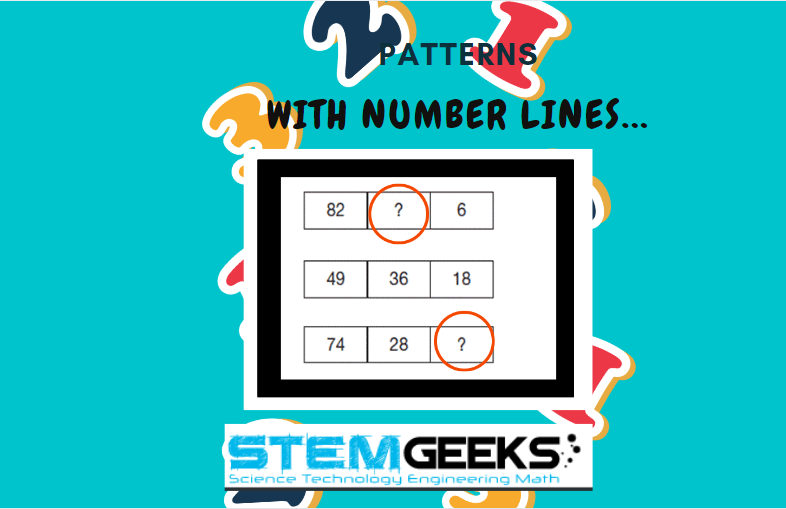With that lets have a look at our Math Brain Teasers 28:: Puzzle - Find You Find The Pattern in Number Lines??? Explore the given number lines in the image above and try finding out the pattern to replace the question marks.

## What I can assure you is that its not that complicated. If you are able to recognize the hidden pattern then it should be easy to solve. Simple Mulitiplication is the hint to solve this Math Brain Teasers 28:: Puzzle

##### Math Brain Teasers 27:: Puzzle - What Should Replace The Question Mark?- Solved with Explanation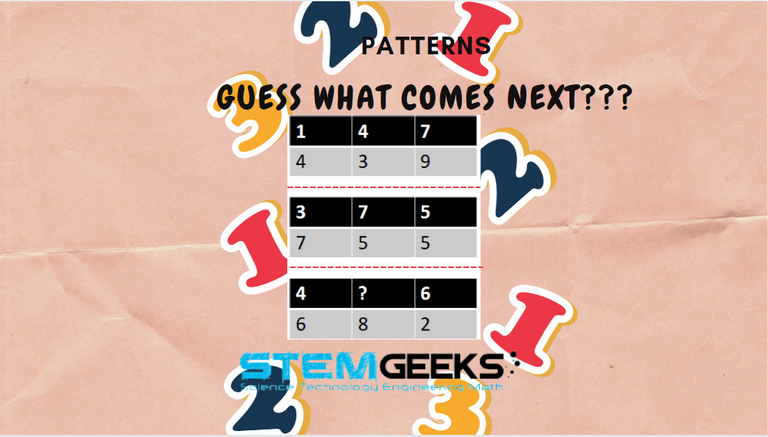Lets us first see how the first table is solved...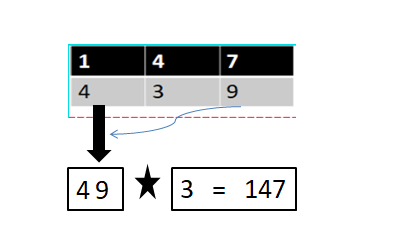### 49 x 3 = 147 and

Similarly for the Second table it will be

### 62 x 8 = 496;

Now the table in question the Answer would be::

### STEM token GiveAway

I'll be again doing a giveaway of STEM tokens to the lucky random winner with the correct answer. For the last contest, which was Math Brain Teasers 27:: Puzzle - What Should Replace The Question Mark?

Surprisngly we didn't have any entry to the puzzle and hence I'll be rolling over the reward i.e. 10 STEM token for Math Brain Teasers 28:: Puzzle

### Math Quote for the Day::

Here is the motivation to solve the Maths Equation Puzzle?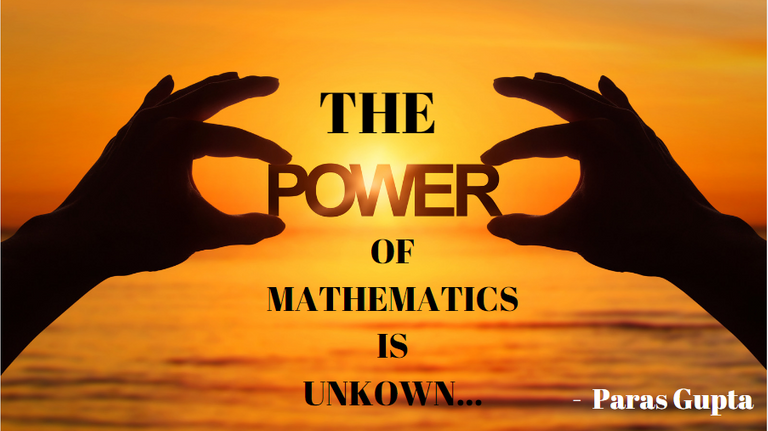If you like my work, then please spread the Word.. that we do have the Math Brain Teasers competition here @StemGeeks platform. Reblog is much appreciated.

Best Regards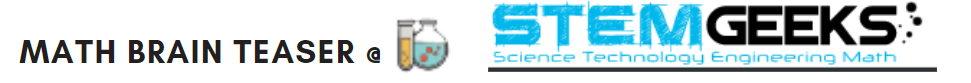Find Me on the Other Social Media Platforms::PS:: All the Maths Brain Teasers; are made by me using the Pro Canva License Version

Sort:

16